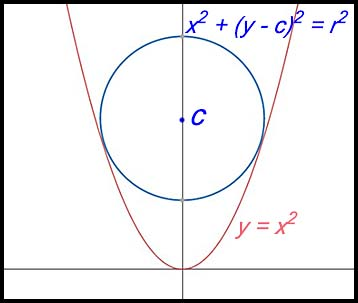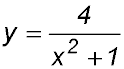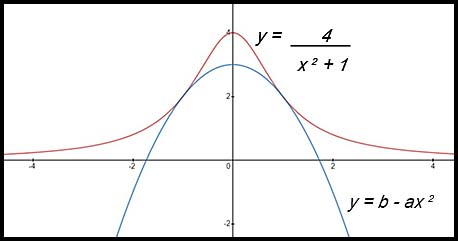Dr. J's Maths.com
Where the techniques of Maths
are explained in simple terms.

 No real roots. 1. Show that the equation x2 + 5x + 8 = 0 has no real roots. 2. Show that the equation 2x2 - 12x + 25 = 0 has no real roots. 3. For what values of p will the quadratic equation x2 + (p - 2)x + 1 = 0 have unreal roots? 4. Explain why the graph of the parabola described by the equation y = 3x2 - 2x + 4 is located above the x axis for all values of x. Real roots 5.For what value of m are the roots of f(x) = mx2 + 6x - 3 real? 6. (i) Find the discriminant of the equation 3x2 + 2k + k = 0. (ii) For what values of k does the equation 3x2 + 2k + k = 0 have real roots? 7. Show that x2 + kx + k - 1 = 0 has real roots for all values of k. 8. One real root 9. Find the value(s) of k for which the quadratic equation 3x2 + 2x + k = 0 has one real root. Answer. k = 1/3. 10. Find the values of k for which the quadratic equation x2 - (k + 4)x + (k + 7) = 0 has equal roots. Answer. k = -4 or k = -8. 11. For what value(s) of k does the equation y = x2 - 2kx + 6k have one real root? Answer. k = 0 or k = 6. 12. Real and different roots. 13. Find the value(s) of k if the roots of the equation x2 + kx + 36 = 0 are real and distinct. 14. 15. For the quadratic equation x2 + (p - 3)x - (2p + 1) = 0 (i) Show that the value of the discriminant can be expressed as p2 + 2p + 13. (ii) Hence or otherwise show that the quadratic equation above will always have real, distinct roots for real valued p. 16. Mixed questions. 17. For what values of k does x2 - (k + 5)x + 9 = 0 have (i) equal roots (ii) no real roots. Positive definite. 19. For what values of k is the equation kx2 - (k + 1) - 2k + 3 = 0 positive definite? 20. For what values of k is the equation (k + 1) x2 - 2(2 + k) + 3 = 0 positive definite? Answer. 4 - 2√6 < k < 4 + 2√6. 21. Negative definite. 23. 25. 26. Advanced contexts. 27. (i) Show that for all values of m, the line y = mx - 3m2 touches the parabola x2 = 12y. (ii) Find the values of m for which this line passes through the point (5,2). (iii) Hence determine the equations of the two tangents to the given parabola from the point (5,2). 28. The circle x2 + (y – c)2 = r2, where c > 0 and r > 0, lies inside the parabola y = x2. The circle touches the parabola at exactly two points located symmetrically on opposite sides of the y-axis, as shown in the diagram.(i) Show that 4c = 1 + 4r2 (ii) Deduce that c > ½. 29. The parabola y = b - ax2 , where a > 0 and b > 0 , is under the curve.The parabola touches the curve at two points that are symmetrical at the y-axis, as shown in the diagram above. Let the two curves intersect at x = ± q. (i) Show aq4 + (a - b)q2 + 4 - b = 0 (ii) Hence show that. (iii) Hence show 0 < a < 4. 30. (i) Write down the discriminant of 2x2 + (k –2)x + 8, where k is a constant. (ii) Hence, or otherwise, find the values of k for which the parabola y =2x2 + kx + 9 does not intersect the line y =2x +1. Increasing functions. 31. For what values of k is the curve y = x3 - 3x2 + kx + 3 always an increasing function? 32. Decreasing functions. 33. 34.